# Lecture Three: Connecting the Dots

by Kirby Urner
Oregon Curriculum Network

First posted: Sept 20, 2002
Last updated: Oct 10, 2002

I. MORE PROGRAMMING AROUND PASCAL'S TRIANGLE

Let's start off with Pascal's Triangle, using a methods from Lecture Two:

```   |: !/~ (i.10) NB. transpose (|:) table of binomials (!/)
1 0  0  0   0   0  0  0 0 0
1 1  0  0   0   0  0  0 0 0
1 2  1  0   0   0  0  0 0 0
1 3  3  1   0   0  0  0 0 0
1 4  6  4   1   0  0  0 0 0
1 5 10 10   5   1  0  0 0 0
1 6 15 20  15   6  1  0 0 0
1 7 21 35  35  21  7  1 0 0
1 8 28 56  70  56 28  8 1 0
1 9 36 84 126 126 84 36 9 1
```

I introduced a tiny wrinkle: ~ after a verb with only a right argument copies it to the left, making the verb dyadic. ~ after a verb with two arguments switches them around:

```    +~ 2        NB.  copy 2 to the other side of +
4
3 - 2
1
3 -~ 2       NB. switch arguments to -
_1
```

Now let's develop Pascal's Triangle in the way we normally introduce it to school children. Typically, it has the form of a triangle:

```       1
1   1
1   2   1
1   3   3   1
...```

The terms in a row may be derived by taking the sum of two terms on the row above, to the immediate left and right. If we're already at the far left or right of the triangle, imagine a 0 for the "missing" term.

When displayed in rectangular form, i.e. as a 0-padded array, the rule for generating the terms of a subsequent row is: take the sum of the term directly above, plus the term to its left. At the left edge of the array, add 1 + 0.

Consider the row 1 3 3 1: how would we add each term to the term immediately preceding it? One idea would be to get all the consecutive pairs (1 3, 3 3, 3 1) and take their sums (4 6 4), and then apply a 1 to each end of the resulting list (1 4 6 4 1). J provides the necessary grouping behavior:

```   2 <\ 1 3 3 1   NB. Box operator applied to groups of 2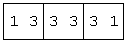2 +/\ 1 3 3 1  NB. monadic add insert (+/) gives sums
4 6 4```

We now have the raw materials we need to generate a subsequent row from a previous one. Let's define separate verbs to accomplish the steps of adding pairs, appending and prepending a 1, then compose all these together using the @ conjunction:

```
sumpairs =: 2 & (+/ \)  NB.  sum groups (bond 2 to +/\)
sumpairs 1 3 3 1
4 6 4

append1 =: ,&1          NB.  bond 1 to catenate (,)
prepend1 =: 1&,         NB.  bond 1 as left argument
nextrow =: append1 @ prepend1 @ sumpairs  NB. long verb

nextrow 1 3 3 1         NB.  test our fancy new verb
1 4 6 4 1

nextrow 1 4 6 4 1       NB.  apply to output of previous
1 5 10 10 5 1

(nextrow ^: 10) 1       NB.  10 applications against 1
1 10 45 120 210 252 210 120 45 10 1

nextrow f.              NB.  force substitutions,&1@(1&,)@(2&(+/\))
```

The last line above uses the force modifier (f.) to replace nextrow's named components with their original definitions. Depending on how J is configured (via the configuration menu shown in the last lecture, or by various commands), the result may be a linear view, as just shown, or a tree view, box view (the default), or a more heavily parenthesized version.

II. VIEWING VERBS

The tree and boxed views may be especially useful when figuring out how a verb breaks down into component actions:

```   nextrow f.   NB. boxed view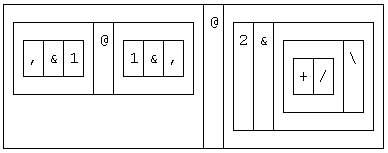nextrow f.   NB. changed configuration to tree view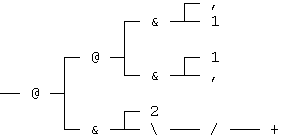```

So we've ended up where we started (by way of much edifying exploration), with a method for generating Pascal's Triangle:

```
] pascal =: (nextrow ^: (i.11)) 1 NB. pascal used below
1  0  0   0   0   0   0   0  0  0 0
1  1  0   0   0   0   0   0  0  0 0
1  2  1   0   0   0   0   0  0  0 0
1  3  3   1   0   0   0   0  0  0 0
1  4  6   4   1   0   0   0  0  0 0
1  5 10  10   5   1   0   0  0  0 0
1  6 15  20  15   6   1   0  0  0 0
1  7 21  35  35  21   7   1  0  0 0
1  8 28  56  70  56  28   8  1  0 0
1  9 36  84 126 126  84  36  9  1 0
1 10 45 120 210 252 210 120 45 10 1
```

III. FIGURATE NUMBERS IN PASCAL'S TRIANGLE

 The vertical columns of pascal are of mathematical interest, not just the horizontal rows (which latter, as we've seen, comprise the binomial coefficients of polynomial (x+1)^n). The 2nd column (column 1 if we index from 0) is clearly the consecutive integers. Less obviously, the next column over (column 2) is the sequence of triangular numbers, equivalently the running totals of the consecutive integers. Indeed, each column is comprised of the running totals of the column to its left.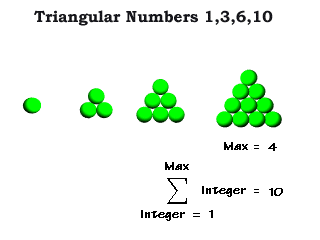We can check this by using \ without a grouping number on the left. This will generate groups with successively more items, starting with 1 item and ending with the entire right argument. This is most easily shown using the boxing operator (<). ``` i.6 0 1 2 3 4 5 &\ i.6 NB. box (<) shows effect of \ adverb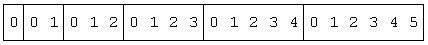+/ \ i.6 NB. running totals of consecutive integers 0 1 3 6 10 15 ``` How shall we extract a single column from pascal? The take verb ({) breaks its argument into items of one less dimension than the argument itself. If the right argument is a two dimensional table, it takes one dimensional rows. Given a 3-dimensional report of multiple tables, it takes tables: ``` ] report =: i. 3 2 2 NB. 0..11 as 3 2x2 tables 0 1 2 3 4 5 6 7 8 9 10 11 1 { report NB. item 1 is a 2x2 table 4 5 6 7``` So taking the nth item of pascal means taking the nth row (starting with row 0 across the top). ``` 1 { pascal NB. take first item (= row) 1 1 0 0 0 0 0 0 0 0 0``` However, we can alter the behavior of { by telling it what dimension of item to eat. By using the rank conjunction (") and specifying a rank (i.e. dimension) of 1, we tell { to start with individual rows of pascal, which entails breaking rows into items of yet one less dimension (in this case into atoms of rank 0) and grabbing the nth such item from each row. ``` 1 {"1 pascal NB. take atom 1 from rank 1 item 0 1 2 3 4 5 6 7 8 9 10 2 {"1 pascal NB. take atom 2 from each row 0 0 1 3 6 10 15 21 28 36 45``` Now we can obtain running totals for a column, and see our result matches the next column to the right: ``` +/\ 2 }"1 pascal NB. running totals of column 2 0 0 1 4 10 20 35 56 84 120 165 +/\ 3 }"1 pascal NB. running totals of column 3 0 0 0 1 5 15 35 70 126 210 330 +/\ 4 }"1 pascal NB. = column 5 of Pascal's Triangle 0 0 0 0 1 6 21 56 126 252 462 ``` Returning to groups of two, notice that pairs of consecutive triangular numbers always add to a square number: ``` triangular =: 2 {"1 pascal NB. extract column 2 2 <\ triangular NB. box the pairs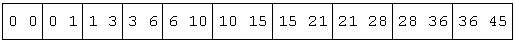] squares =: 2 +/\ triangular NB. sum the pairs0 1 4 9 16 25 36 49 64 81 ```
 Running totals of triangular numbers has an easy geometric interpretation by the way: we're looking at successively larger tetrahedra made of triangles stacked atop each other -- oranges in a grocery store are often stacked in this way. Likewise, running totals of square numbers would give a growing half-octahedron, or square-based pyramid: ``` +/ \ squares NB. half-octahedral numbers 0 1 5 14 30 55 91 140 204 285 ``` Oranges often appear in this form too, and as a matter of fact, both are forms of the same packing, cubic close packing (CCP). Another way to visualize the CCP is of successive shells of balls growing around a nucleus, and taking the shape of a cuboctahedron. Seen this way, the half-octahedral and tetrahedral aspects of the packing are both evident at once.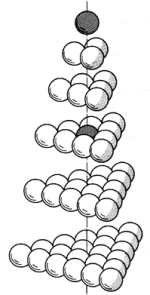Adapted from Fig 419.30 in Synergetics by RBF
 IV. CUBOCTAHEDRAL NUMBERS In the case of the cuboctahedron, the number of balls in successive shells is 10 F^2 + 2, where F = 1,2,3... is the frequency of the shell, i.e. number of between-ball intervals along an edge. The cuboctahedral numbers would be running totals of the shells, plus the nuclear ball at the center. ``` shell=: (2&+) @ (10&* @ *:) NB. 2 + 10* F^2 shell >:i.10 NB. F = 1,2,3... 12 42 92 162 252 362 492 642 812 1002 ] cuboctahedral =: 1,1 + +/\ shell >:i.10 NB. add nuke 1 13 55 147 309 561 923 1415 2057 2869 3871``` The above series may be expressed more succinctly by evaluating the polynomial (10/3)n^3 - 5n^2 + (11/3)n - 1, where n = 1,2,3... Let's see this using rational number coefficients along with the polynomial operator introduced in the previous lecture: ``` poly =: _1 11r3 _5 10r3 & p. ] cuboctahedral =: poly >:i.11 1 13 55 147 309 561 923 1415 2057 2869 3871 ``` The main reason for introducing the shells-based approach first, along with the concept of frequency, was to make the link to geodesic spheres. Geodesic spheres are often icosahedrally based, having 12 pentagonal hubs (vertices). Their frequency is the number of struts (edges, intervals) between one pentagonal hub and the next. An icosahedral shell of frequency F has the same number of balls (vertices, hubs) as the corresponding cuboctahedral shell of the same frequency. Why this should be the case is dramatized visually by what R. Buckminster Fuller dubbed the "jitterbug transformation" whereby a cuboctahedral shell may transform into an icosahedral shell and vice versa.Fig. 466.00a from RBF's Synergetics The jitterbug transformation takes us from a polyhedron with 3 and 4-fold rotational symmetry (the cuboctahedron), to another polyhedron of five-fold rotational symmetry (the icosahedron). Now that we've "jitterbugged" into the domain of five-fold rotational symmetry, by distorting one of the cuboctahedron's shells, we may suppose that we've left Pascal's Triangle behind, yet it comes up again in an interesting way, in connection with the pentagon, the hallmark of this five-fold world. V. FIBONACCI NUMBERS AND THE GOLDEN MEAN Recall that summing the terms of Pascal's Triangles along oblique diagonals gives us the Fibonacci numbers. ``` (i.11) { +/ /. pascal NB. items 0,1..10 of oblique sums 1 1 2 3 5 8 13 21 34 55 89 11 {. +/ /. pascal NB. 11 {. gets the same thing 1 1 2 3 5 8 13 21 34 55 89 pascal =: (nextrow ^: (i.21)) 1 NB. a bigger triangle fibos =: 21 {. +/ /. pascal NB. more fibonacci numbers fibos = x: fibos NB. convert to rational numbers (x:)``` Taking the Fibonacci numbers in successive pairs, and dividing the larger by the smaller, moves use ever closer to the golden ratio, known to the greeks as phi, also equivalent to (1 + sqrt(5))/2. That the Fibonacci numbers should converge to phi makes sense. 34 is to 55 in about the same way 55 is to the whole (34 + 55 = 89), and this whole is also the next Fibonacci number, and so on. This relationship (of parts to wholes) gets more exact the further out we go. ``` (1+%:5)%2 NB. phi =: (1 + sqrt(5)/2 1.61803 55%34 1.61765 89%55 1.61818``` Phi is defined as a parts-to-whole relationship. Phi is the ratio of two segments B, A such that the smaller (A) is to the larger (B), as the larger (B) is to both combined (A+B). In other words, given A:B = B:(A+B) then if A = 1, B = phi. The quantity (1 + sqrt(5))/2 may be computed directly from this equation. In the section below, we study this convergence to phi, using a couple more tricks around rational numbers and displayed precision (explained below). This section uses the variable fibos defined above: the first 20 Fibonacci numbers stored in rational form. ``` ratios =: 2&(((1&{) % (0&{))\) NB. extract and divide ratios NB. divide bigger by smaller in each group of 2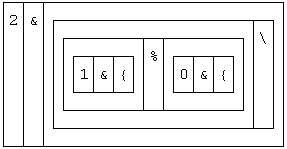5 4 \$ ratios fibos NB. shape ratios into a 5x4 table 1 2 3r2 5r3 8r5 13r8 21r13 34r21 55r34 89r55 144r89 233r144 377r233 610r377 987r610 1597r9872584r1597 4181r2584 6765r4181 10946r6765 (9!:11) 12 NB. !: invokes a "foreign" service (ouch) (x:^:_1) 5 4 \$ ratios fibos NB. convert to decimals 1 2 1.5 1.66666666667 1.6 1.625 1.61538461538 1.61904761905 1.61764705882 1.61818181818 1.61797752809 1.61805555556 1.61802575107 1.61803713528 1.61803278689 1.61803444782 1.6180338134 1.61803405573 1.61803396317 1.61803399852 ] phi =: 1r2 * 1 + x:%:5 NB. phi as rational number phi 657194919625r406168797562 NB. an approximation x:^:_1 phi NB. the decimal version1.61803398875``` VI. INVERSE AND FOREIGN FUNCTIONS We looked at x: in Lecture One, and we know that ^:n is used to apply a function n times. What we haven't seen before is getting an inverse function by applying a function ^:_1 times. For example, the inverse of squaring (*:) is the square root (%:), and the powering operator makes this clear. ``` square =: *: NB. name J's squaring verb squareroot =: (square ^:_1) NB. same as %: square 10 100 squareroot 100 10 ``` Consistently, therefore, the inverse of x: is x:^:_1, and its behavior is to convert a rational into a decimal. Another feature of J is the large library of "foreign" verbs, external to the language proper, and organized into numbered families invoked with the dyadic conjuction !:. These foreign verbs are used to read/write files, convert between data types, get system time, memory statistics, or to toggle the display from box view to tree view (something we've been doing via the GUI -- but could just as well have done on the command line). VI. PLOTTING A PENTAGON Returning to our geometric explorations, the golden ratio (phi) describes the ratio of any of the regular pentagon's five edges to any of the pentagon's diagonals, which in turn describe a five-pointed star. We can show this graphically, and then obtain phi by taking the ratio between a pentagon edge and a star edge. The technique we use recaps what we did at the end of Lecture One: ``` (9!:11) 6 NB. display 6 digits of precision rotate =: 1ad72 & * NB. 360 degrees divides into 5 x 72 ] points =: +. rotate ^:(i.6) 1j0 1 0 0.309017 0.951057 _0.809017 0.587785 _0.809017 _0.587785 0.309017 _0.951057 1 _2.22045e_16 pentx =: 0{"1 points NB. grab first column (x coords) penty =: 1{"1 points NB. grab 2nd column (=y coords pentagon =: pentx; penty load 'plot' plot pentagon starx =: 0 2 4 1 3 0 { 0{"1 points NB. reordered points stary =: 0 2 4 1 3 0 { 1{"1 points star =: starx; stary plot star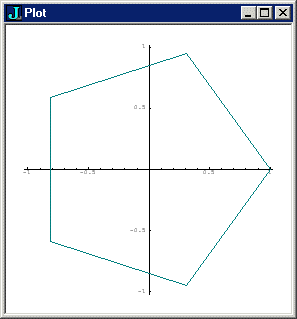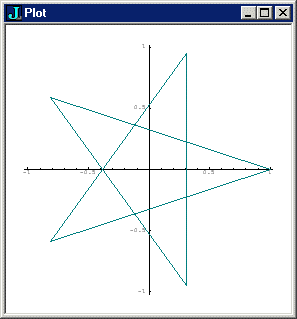'A B C' =: 0 1 2 { points NB. take 3 points A,B,C distance =: %: @ +/ @: (*: @ -) NB. distance formula ``` Finally, I should explain this mystery of @: versus @ (@ inflected with a colon, vs. used alone). Let's look at this distance formula in detail. On the far right, we encounter the pattern (f@g), which, in a dyadic situation, translates to f(g(x,y)). Remember our table from Lecture Two: ```g(x,y) <==> x g y NB. simple dyadic form f(g(x,y)) <==> x (f @ g) y NB. dyadic g works on x,y) ``` So the subtraction operator gets used dyadically to subtract B from A, and these are both 2-element arrays (x and y coordinates), so the result is also a 2-element array. ``` A 1 0 B 0.309016994375 0.951056516295 A-B 0.690983005625 _0.951056516295``` *: then squares both (Ax-Bx) and (Ay-By) -- all a standard part of computing distance. Notice that this technique would work just as well for 3-dimensional coordinates, or more. ``` *: A-B 0.477457514063 0.904508497187``` Now comes the interesting part. If +/ were glued to the squaring verb *: using @, it would follow "atop" the squaring verb, tightly coupled to it, applying immediately to each squared item. But what we want is to apply +/ to the entire row, i.e. we want *: to get through with its business and then use +/ to insert + between its elements. This is where @: comes in: it says "allow the action on the right to build up to as many dimensions as necessary, and then eat it as a single whole" -- and in this case, "whole" means a 1-dimensional array of numbers. ``` +/ @ *: A-B NB. +/ applies to each squared atom 0.477457514063 0.904508497187 +/ @: *: A-B NB +/ applies to item of rank 1 (row) 1.38196601125``` So the summation verb + adverb (+/) adds both squared differences (or more, e.g. if we're in a 3-dimensional coordinate system), giving back a single number, of which *: then takes the 2nd root. So now we have our distances. But do we have phi? ``` (9!:11) 12 NB. go back to 12 digit display (A distance C) % (A distance B) NB. ratio = phi 1.61803398875``` VII. PHI AS A CONTINUED FRACTION Phi is also arguably the simplest of the non-terminating continued fractions, with all coefficients = 1. J makes it easy to do continued fractions, given its right-to-left execution mode. The conventional expression: ` 1 + 1 /( 1 + 1 /(1 + 1 /(1 + 1/(1 + (1 + 1/(1 + 1/1)))))) ` is rewritten as: `(+ %) / 1 1 1 1 1 1 1` a hook which generatates `1 + %(1 + %(1 + %(1 + %(1 + %(1 + %1))))):` To summarize: ``` 1+1%(1+1%(1+1%(1+1%(1+(1+1%(1+1%1)))))) NB. dyadic % 1.63158 1 + %(1 + %(1 + %(1 + %(1 + %(1 + %1))))) NB. monadic % 1.61538 ( + %)/ 1 1 1 1 1 1 1 NB. hook: (+ %) 1 = 1 + %1 1.61538 (+ %) / 1 1 1 1 1 1 1 1 1 1 1 NB. approximating phi 1.61797752809 (+ %) / 20#1 NB. apply to a list of 20 1s 1.61803399852 (+ %) / 40#1 NB. 40#1 makes "40 copies of 1" 1.61803398875 x:^:_1 phi NB. and there we have it!1.61803398875 ``` For further reading: Official J website Jiving in J Introductory J Lectures:  Trends in Early Math Education CP4E